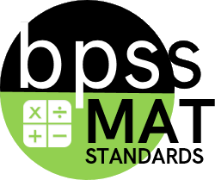(NBT) Number and Operations in Base Ten Categories All categories Not categorized Prioritized (G) Geometry (MD) Measurement and Data (NBT) Number and Operations in Base Ten (NF) Number and Operations - Fractions (OA) Operations and Algebraic Thinking

# Number and Operation in Base Ten

## Narrative for the (NBT) Number and Operation in Base Ten

At Grade 4, students extend their work in the base-ten system. They use standard algorithms to fluently add and subtract. They use methods based on place value and properties of operations supported by suitable representations to multiply and divide with multi-digit numbers. Visual representations such as area and array diagrams that students draw and connect to equations and other written numerical work are useful for this purpose. By reasoning repeatedly about the connection between math drawings and written numerical work, students can come to see multiplication and division algorithms as abbreviations or summaries of their reasoning about quantities.

Building on earlier work, students generalize place value understanding for multi-digit whole numbers to read, write, and compare whole numerals between 1,000 and 1,000,000. They also extend understanding of place value to include decimals to hundredths and fractional quantities 1/10 and 1/100.

## Calculation Method for Domains

Domains are larger groups of related standards. The Domain Grade is a calculation of all the related standards. Click on the standard name below each Domain to access the learning targets and rubrics/ proficiency scales for individual standards within the domain.

#### MAT-04.NBT.01

Under Development

 MAT-04 Targeted Standards(NBT) Domain: Number and Operations in Base TenCluster: Generalize place value understanding for multi­digit whole numbers. Limited to whole numbers less than or equal to 1,000,000. MAT-04.NBT.01 Recognize that in a multi-digit whole number, a digit in one place represents ten times what it represents in the place to its right. For example, recognize that 700 ÷ 70 = 10 by applying concepts of place value and division.

• I can

• I can

• I can

• I can

## Proficiency (Rubric) Scale

 Score Description Sample Activity 4.0 Student is able to - 3.5 In addition to Score 3.0 performance, the student demonstrates in-depth inferences and applications regarding the more complex content with partial success. 3.0 “The Standard.” Student is able to - 2.5 No major errors or emissions regarding 2.0 content and partial knowledge of the 3.0 content. 2.0 Student is able to - 1.5 In addition to 1.0 content, student has partial knowledge of the 2.0 and/or 3.0 content. 1.0 Student is able to - 0.5 Limited or no understanding of the skill id demonstrated.

## Resources

### Vocabulary

• List

#### MAT-04.NBT.024th Grade (MAT) Targeted Standard
(NBT) Number and Operations in Base Ten
Cluster: Generalize place value understanding for multi­digit whole numbers. Limited to whole numbers less than or equal to 1,000,000.

#### MAT-04.NBT.03

Under Development

 MAT-04 Targeted Standards(NBT) Domain: Number and Operations in Base TenCluster: Generalize place value understanding for multi­digit whole numbers. MAT-04.NBT.03 Use place value understanding to round multi-digit whole numbers to any place.

• I can

• I can

• I can

• I can

## Proficiency (Rubric) Scale

 Score Description Sample Activity 4.0 Student is able to - 3.5 In addition to Score 3.0 performance, the student demonstrates in-depth inferences and applications regarding the more complex content with partial success. 3.0 “The Standard.” Student is able to - 2.5 No major errors or emissions regarding 2.0 content and partial knowledge of the 3.0 content. 2.0 Student is able to - 1.5 In addition to 1.0 content, student has partial knowledge of the 2.0 and/or 3.0 content. 1.0 Student is able to - 0.5 Limited or no understanding of the skill id demonstrated.

## Resources

### Vocabulary

• List

#### MAT-04.NBT.044th Grade (MAT) Targeted Standard
(NBT) Number and Operations in Base Ten
Cluster: Use place value understanding and properties of operations to perform multi-­digit arithmetic.

#### MAT-04.NBT.054th Grade (MAT) Targeted Standard
(NBT) Number and Operations in Base Ten
Cluster: Use place value understanding and properties of operations to perform multi­-digit arithmetic.

#### MAT-04.NBT.064th Grade (MAT) Targeted Standard
(NBT) Number and Operations in Base Ten
Cluster: Use place value understanding and properties of operations to perform multi­-digit arithmetic.